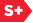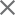### Proceedings Paper

A proposal of the diagnosis-dynamic characteristic (DDC) model describing the relation between search time and confidence levels for a dichotomous judgment, and its application to ROC curve generation
Author(s): Toru Matsumoto; Nobuo Fukuda; Akira Furukawa; Koji Suwa; Shinichi Wada; Mitsuomi Matsumoto; Shusuke Sone
Format Member Price Non-Member Price
PDF \$17.00 \$21.00

Paper Abstract

When physicians inspect an image, they make up a certain degree of confidence that the image are abnormal; p(t), or normal; n(t)[n(t)=1-p(t)]. After infinite time of the inspection, they reach the equilibrium levels of the confidence of p*=p(∞) and n*=n(∞). There are psychological conflicts between the decisions of normal and abnormal. We assume that the decision of "normal" is distracted by the decision of "abnormal" by a factor of k(1 + ap), and in an inverse direction by a factor of k(1 + bn), where k ( > 0) is a parameter that relates with image quality and skill of the physicians, and a and b are unknown constants. After the infinite time of inspection, the conflict reaches the equilibrium, which satisfies the equation, k(1 + ap*)n* = k(1 + bn*)p*. Here we define a parameter C, which is 2p*/[p*(1 - p*)]. After the infinite time of inspection, the conflict reaches the equilibrium, which satisfies t that changes in the confidence level with the time (dp/dt) is proportional to [k(1+ap)n - k(1+bn)p], i.e. k[-cp2 + (c - 2)p + 1]. Solving the differential equation, we derived the equation; t(p) and p(t) depending with the parameters; k, c, S. S (0-1) is the value arbitrary selected and related with probability of "abnormal" before the image inspection (S = p(0)). Image reading studies were executed for CT images. ROC curves were generated both by the traditional 4-step score-based method and by the confidence level; p estimated from the equation t(p) of the DDC model using observed judgment time. It was concluded that ROC curves could be generated by measuring time for dichotomous judgment without the subjective scores of diagnostic confidence and applying the DDC model.

Paper Details

Date Published: 17 March 2006
PDF: 14 pages
Proc. SPIE 6146, Medical Imaging 2006: Image Perception, Observer Performance, and Technology Assessment, 61460Y (17 March 2006); doi: 10.1117/12.652933
Show Author Affiliations
Toru Matsumoto, National Institute of Radiological Sciences (Japan)
Nobuo Fukuda, National Institute of Radiological Sciences (Japan)
Akira Furukawa, National Institute of Radiological Sciences (Japan)
Koji Suwa, Nippon Dental Univ. (Japan)
Mitsuomi Matsumoto, Diichi Hospital (Japan)
Shusuke Sone, JA Azumi General Hospital (Japan)

Published in SPIE Proceedings Vol. 6146:
Medical Imaging 2006: Image Perception, Observer Performance, and Technology Assessment
Yulei Jiang; Miguel P. Eckstein, Editor(s)